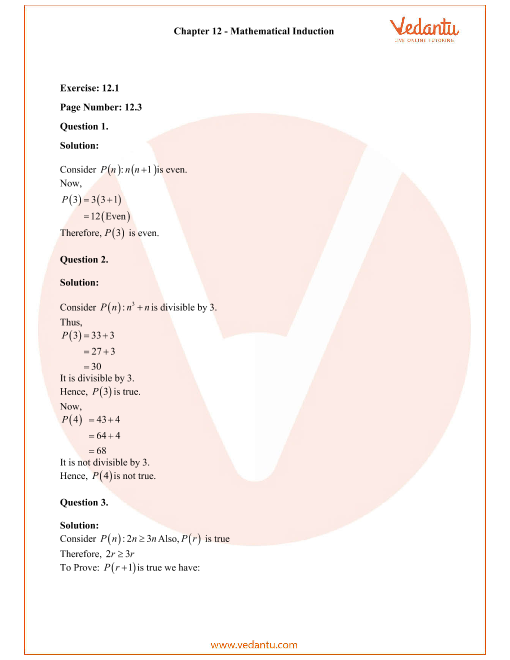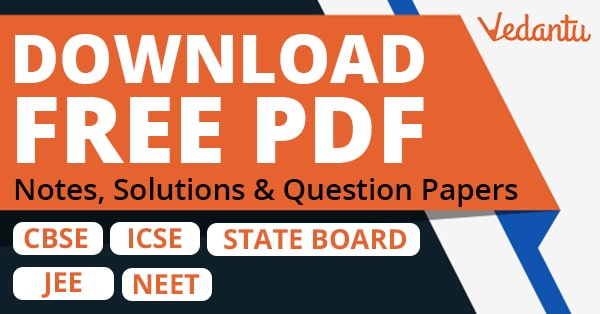RD Sharma Class 11 Solutions Chapter 12 - Mathematical Induction (Ex 12.1) Exercise 12.1

RD Sharma Class 11 Solutions Chapter 12 - Mathematical Induction (Ex 12.1) Exercise 12.1 - Free PDF

Free PDF download of RD Sharma Class 11 Solutions Chapter 12 - Mathematical Induction Exercise 12.1 solved by Expert Mathematics Teachers on Vedantu.com. All Chapter 12 - Mathematical Induction Ex 12.1 Questions with Solutions for RD Sharma Class 11 Maths to help you to revise complete Syllabus and Score More marks. Register for online coaching for IIT JEE (Mains & Advanced) and other Engineering entrance exams.